Browse by Topic
Related Topics

# Mathematica Q&A: Three Functions for Computing Derivatives

Got questions about Mathematica? The Wolfram Blog has answers! We’ll regularly answer selected questions from users around the web. You can submit your question directly to the Q&A Team using this form.

This week’s question comes from Bashir, a student:

What are the different functions for computing derivatives in Mathematica?

The main function for computing derivatives in Mathematica is D, which computes the familiar partial derivative of an expression with respect to a variable: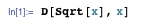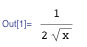D supports generalizations including multiple derivatives and derivatives with respect to multiple variables, such as differentiating twice with respect to x, then once with respect to y: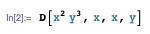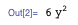And vector and tensor derivatives, such as the gradient: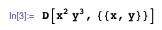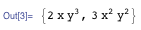There are two important properties of D[expr, x] that distinguish it from other functions for computing derivatives in Mathematica:

1. D computes the derivative of an expression representing a quantity, such as Sin[x], not a function, such as Sin. Compare with Derivative below.

2. D takes the partial derivative to be zero for all subexpressions that don’t explicitly depend on x. Compare with Dt below.

To differentiate a function, you use Derivative, which has the standard shorthand notation ' (apostrophe):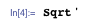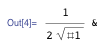The result is a pure Function of one unnamed argument #1. Note that if you immediately evaluate this function at x, the result is exactly what you would have found by using D to differentiate the quantity Sqrt[x] with respect to x: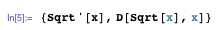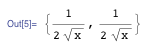The notation f' is shorthand for Derivative[f], specifying differentiation once with respect to the first argument. As with D, generalizations like multiple derivatives and derivatives with respect to multiple variables are possible: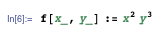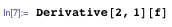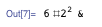Derivative[2, 1] specifies differentiation twice with respect to the first argument and once with respect to the second argument. (You could also write the above in a single line as Derivative[2, 1][#1^2 #2^3&].)

Dt[expr, x] computes the total derivative of the expression expr with respect to x. It works like D[expr, e], except Dt does not assume zero derivative for parts of expr with no dependence on x. Compare D and Dt in this short example:D assumes a is a constant independent of x; Dt does not, and Dt[a, x] remains unevaluated.

This can be useful in situations where you have variables that implicitly depend on some other variable. For example, suppose you want the time derivative of x + y z given in terms of the time derivatives of x, y, and z. You can use Dt:To do this with D, you would explicitly make x, y, and z functions of t:Finally, the one-argument form Dt[expr] gives the total differential of the expression expr: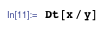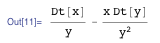The result is given in terms of the differentials (Dt[x], Dt[y], in this case) of the variables occurring in expr.

When viewed in traditional mathematical notation (TraditionalForm), this example looks familiar as the standard quotient rule for differential quantities: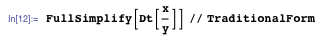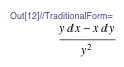If you have a question you’d like answered in this blog, you can submit it to the Q&A Team using this form. For daily bite-sized Mathematica tips, follow our @MathematicaTip Twitter feed (or follow using RSS).